The Work that is Created by Means of Potential Field

Report on the International Conference "New Ideas in Natural Sciences",
St.-Petersburg, June 1996

Alexander V. Frolov
Scientific Expert of Russian Physical Society
P.O.Box 37, 193024, St.-Petersburg, Russia

Introduction

The concept for physical vacuum as energy source is proved mathematically . It is recognized that space itself have inner structure and it can be used as source of energy if some process is organized to change the structure of space. The existence of reality is described by means of density probability function for energy . So, any space is result of some energy process and there is no space that have not energy at all. Some power process can be designed in any point of the space by means of energy transformation.

Some Definitions

1. Potential  (lat. potentia, that means "force" ) is possibility to make some action, to make some work. In physics it is scalar parameter. Gradient of potential is intensity of some field.
2. Field is area of action for some force.
3. Force is reason ( cause ) for motion.
4. Work is quantitative description for energy transformation. Quantity of work per unit of time is power.
4. Energy ( Greek - "energie" means "action" ) is quantitative description for different forms of motion.

Power Extraction in the Point of Changing Potential

The potential j=max on the charged mass M surface and potential is equal to zero j=0
for infinity distance from mass M. There is some gradient and intensity of field

E = - grad j                                           F.1

as result of change for potential value in the process of motion from mass M to infinity.

The electric pressure U is known as potential difference  between two points in space,

U = jmax - jmin = Dj                     F.2

But process for measurement of  the pressure is motion from point A to point B in space, and mathematically this work to move the electrically charged mass is derivation procedure:

A = Dj/Dx = j'(x)                               F.3

The work and the power is result of this motion, since potential gradient  means some changes of energy value ( energy transformation ).

For equipotential area case,  any point of space have potential j = const, and in this case there is no changes of energy for measurement in different points of equipotential area. Let's suppose that this area have zero size and it is some point C. In this case there is only one way for motion and energy value changes: it is motion in time. It is possible to consider some motion from the moment CA to the moment CB. If the potential jA(t) is not equal to potential jB(t), there is some gradient of the potential in the point C. But it is not  direction in space but gradient for direction in time, the chronal gradient. This potential difference can be named as chronal pressure.

To note this difference  is used  designation "gradj(t)" for chronal gradient. Some intensity is created also:

Intensity of the chronal field in the point C is created if potential is function of  time. The change of energy ( transformation of energy ) create the work and in strength of this reason chronal field of one point of space can be used as power source to produce the motion. The motion here is motion in time.

The work is depend of chronal pressure and it is determined as derivation on time:

A = Dj/Dt = j'(t)                     F.5

It is the work to move electrically charged mass in time dimension.

Technical system for power extraction in this case must include the "memory unit" to make the comparison between past potential value jA(t) and future value jB(t). Example for it is the electric capacitor, Fig.1.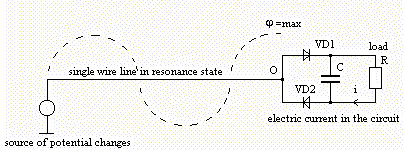Figure 1

The generator of changing potential is connected to the wire. Length of the wire must be corresponding to some resonance value. In resonance case potential changes in the point O are maximum. N.Tesla used special coil to find the resonance state in his single-wire power transmission system.

Special method to get the asymmetry in oscillation of potential here is so call "Avramenko's plug".  It is two diodes connected to common point of changing potential.  Diodes create unidirectional  displacement of the electrons in the wires of this circuit when the potential is changing. Capacitor
here is charging by means of potential that is not influence to the primary power source.  Generator here  is  the source of information only.

Note, that potential value can be alternating or pulsating. The frequency and amplitude of changes determine the power. Also the number of electrons in the wire is important for the current in load.

By analogy with electromotive force EMF that is produced by any potential difference source,
let us introduce into consideration so call chronomotive force CMF. The field of this force is not spatial but chronal. Result of this force action to any mass object is the motion in time.

Permanent CMF in certain point is result of unidirectional change of the potential as function of time. The nature of mass demonstrate the unidirectional motion in time from past to future. By the theory that is proposed here, it is result of some unidirectional global change of certain potential value. This change is global since the effect (so call natural time flow) is detected in all points of  our space-time. Unidirectional change of potential value, for example, electrical, can be used to  produce local changes of the time rate that should be demonstrated as acceleration or deceleration of any process of matter.

So, two methods for creation of energy changes ( in space and in time ) are corresponding to
two methods for power extraction:
1.  By means of closed in space circuit "source - load".
2.  By means of closed in time circuit "cause - effect".

Tesla's Concept for Wireless Power Transmission

By Tesla's papers  the concept for wireless power transmission require the source of high frequency potential electric field. Value of potential he used was a very high also. There is the difference in principle for Tesla's scheme and Hertz's radio transmitter. Fig.2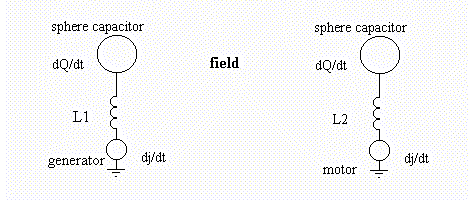Figure 2

Generator G produce changes of the current density dj/dt in wire of the coil and dQ/dt on surface of the sphere capacitor C1. This capacitor is the source of oscillating electric potential field dj/dt (instead of electromagnetic waves by Hertz). It produce longitudinal wave of energy density. Then the changes of induced charge dQ/dt that is created on surface of capacitor C2 is the reason for electric current dj/dt in the wires of the motor and in strength of this reason some power is created in the load  free of any power input. N.Tesla has demonstrated it. More 60 years ago he wrote: " I showed that the universal medium is a gaseous body in which only longitudinal pulses can be propagated, involving alternating compressions and expansions similar to those produced by sound waves in the air. Thus, a wireless transmitter does not Hertz waves which are a myth, but sound waves in the ether, behaving in every respect like those in the air, except that, owing to the great elastic force and extremely small density of the medium, their speed is that of light." It is part of N.Tesla's article "Pioneer Radio Engineer Gives Views on Power", published in  New York Herald Tribune, Sept. 11, 1932, [7, p.94].  Tesla wrote about great mistake of modern science: "The Hertz wave theory of wireless transmission may be kept up for a while, but I do not hesitate to say that in a short time it will recognized as one of most remarkable and inexplicable aberrations of the scientific mind which has ever been recorded in history", article "The True Wireless" [ 7, p.95 ].
So, there is no directed radio beam here. In the resonance secondary terminal C2 can produce free power in load that is equal to oscillating generator power. Also several secondary terminals can be used for power extraction by means of changing potential field.

Electrolysis as Work of Potential Field to Move the Ions

In 1888 Russian scientist Dmitry A. Latchinov, inventor of electrolytic way for hydrogen production, has patented high efficient way for electrolysis of water. He demonstrated the paradoxical situation: electrolytic cell produced high pressure gas but it required the same input power electricity that was used for low pressure gas production. Secondary effect for this high pressure gas case is self-cooling of the electrolytic cell since it need to compensate excess power output by means of its inner heat energy. It was in contradiction with second law of the thermodynamic but now there are many explanations for heat-to-work energy conversion.

It was real example for heat pump technology that is connected with syntropy but not with entropy function. Physically the power generation mechanism here is the work of potential field between electrodes to move the ions. Electrical current from primary source is not the necessary condition for electrolysis. Only potential field produce the decomposition of water and power output can be much more than power is used for potential field creation.

Note: entropy function for work-to-heat energy transformation is correspond to normal rate of time flow from past to future. It is possible to assume the correlation between syntropy function of some power generation process by means heat-to-work conversion and reversed time direction.

Acceleration in Potential Field

In the classical example the potential  field make work to accelerate the body (acceleration  a>0 ) but then system have to work to return the body in start point ( acceleration a<0 ). Classical conclusion is: total net work of potential field is equal to zero and potential field can not be used to create the power in load. It is not mistake but it is particular case only.

When parameters of the field or parameters of the working body are changing  it is possible to create only acceleration of the body in potential field.  Examples: Screening for part of the rotor trajectory to use Coulomb forces only for acceleration of the rotor, patent USA 4897592. Another way to exclude negative part of the work is spatial superposition for field source and working body, Fig.3.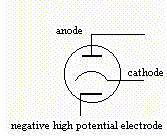Figure 3

Grid electrode that is connected to negative high potential source is placed near cathode. In strength of this reason the field of this electrode make the work to accelerate free electrons are emitted from cathode. So, kinetic energy of the electrons is increasing in the process of the motion of the electrons. Theoretically, power output from anode is more that power input.

Some analogy allow to consider well-known Alexander V. Chernetsky's self-generating discharge as demonstration of the potential field work. Small demonstration device scheme that was made to demonstrate it in the process of the conference New Ideas in Natural Sciences, 17 -22 June 1996, St.-Petersburg, Russia, is shown as Fig.4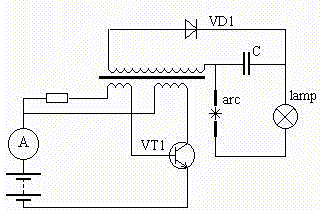Fig.4
Load is connected in serial with discharge arc. When the distance between electrodes is more that minimum arc distance and there is no discharge, there is no current in load ( lamp ) also. The amperemeter ( maximum value is 1 A ) show current 0.3 A. Power input is equal to 10Vx0.3A=3W.  When the arc discharge between electrodes is created, the lamp is lighting and there is the power output about 2 W. But there is no any increase of the input direct current. Amperemeter value is 0.28A that means the decrease of input current when the arc is working in secondary circuit. When  screw-driver make short connection between electrodes the current is equal to 0.58 A. It is ordinary case for any transformator: direct connection of the load produce the increase of consumed power.

Alexander Chernetsky   explained his result as pinch-effect for current of arc that must be equal to many hundred Ampere. In his explanation was used the virtual particles concept and vacuum zero point energy theory.

Other explanation is proposed here. It is clear that charged particle are getting some additional kinetic energy in the process of motion, since it is the accelerated motion in electric field between electrodes.

Note, that Dr. Chernetsky demonstrated in 1980 - 1990 up to 500 Kw output power by means of his self-generation electric discharge method. There are many modern patents on it, for example: U.S. patents numbers 5416391 and 5449989, Paulo Correa, Canada.

Magnetic Field Changes

Free source of the field (field is area of action for some force that we have to use) is permanent magnet. To make some work periodically by means of this constant field it is necessary to create modulation of the flux. Figure 5 is scheme that is very close to USA patent 3879622, John W. Ecklin.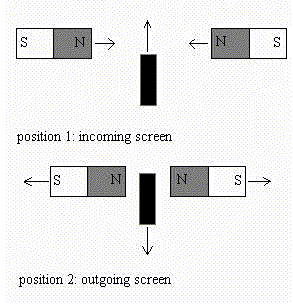Fig.5
Moving metal part periodically change the structure of field in the area between magnets. It is the reason for periodical force interaction that create the power in output. There is no power input to move metal part since it is moving into the field area with some acceleration and it is removing from the field with equivalent deceleration. But magnets and can produce the power free of input.
Other way is rotor that is placing periodically the metal part in the area between magnet and coil of the generator to create alternating field instead of permanent field. It is so called alternator.

So, output power is free if the permanent magnet field source is used to make the changes of  energy density in the point of the power extraction.

Inner Structure of Potential Field

The Whittaker's potential concept is considering the potential as bi-directional energy flow . Thomas E. Bearden  wrote in his book Gravitobiology, p.2:  "Space-time in a sense may be regarded as a conglomerate of potentials - including the scalar EM potentials. Therefore the simplest structure of EM scalar potentials (trapped EM energy) is also nominally composed of such spin-2 gravitons." Structure of graviton and potentials, by Bearden, is coupled photon/antiphoton pair. Antiphoton is reverse-time process. Therefore, the potential field can be considered as a bi-directional electromagnetic process/antiprocess.
So, the power can be produced by means of potential field only as inner field structure disbalance. Bidirectional energy flow described above, mathematically can be presented as the equation

0 = A + B                                                           F.6

where zero balance is created by means of two opposite process. If one of the processes, for example, A is the source of power ( zero-point vacuum electromagnetic as power source ), so according to F.4 other part of the balanced system that is process B should be changed also.

So, for  such sort process there is equation

0 = dA/dt + dB/dt                                                 F.7

and in other view

dA/dt = - dB/dt                                                     F.8

If time for B part is considered as reversed time tr  and time for process A is considered as direct time td , we obtain the equation for total energy conservation law that is taking into consideration the direct time and reversed time energy processes

dA/dtd = dB/dtr                                                      F.9

Conclusion by F.7: Space power is unlimited.

There is no limit for power extraction if change of value A is correspond to change of value B.

The conservation law for energy means the conservation of time balance:

The energy of all processes in direct time must be equal to the Energy of all processes in reversed time.

This formulation  is unification of Newton mechanics law (action - reaction ) and conservation law. In general view it can be named as balance law.

Note that zero is not nothing. It is balanced  structure. It is known from ancient mathematics. In general case it is possible to consider the many-polarity for energy balance:

0 = A + B + C + ... + N                                                F.10

Time structure can be more complex than bi-directional "time/reverse-time" and the chronal area like space area can be considered in many-dimensional version also.

Cause and Two Contra-directional Effects for Spatial Separation

Simple example for two spatially separated effects are operating from one cause is this magnet device, Fig. 6.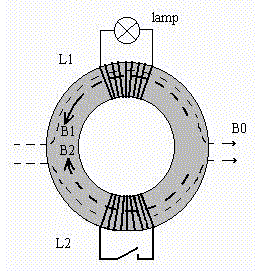Fig.6
It is closed in loop ferromagnetic core and two coils L1 and L2. Let's  assume that primary flux is increasing.  Direction of secondary induced flux B1 in area of coil L2 is co-directional to primary flux. Also direction of secondary induced flux B2 in area of coil L1 is co-directional to primary flux. So, secondary fluxes are contra-directional to each other.
If primary flux is not increasing but decreasing the situation is the same. So,  from the primary source point of view the secondary fluxes are mutual-compensated. It means that two power sources L1 and L2 are working without any power consumption.

Demonstration system for the conference included several permanent magnets are placed inside of disk plastic rotor, that is rotating by small electromotor. In experiments it was demonstrated that power in load of L1 is increasing when coil L2 is connected.

So, when two contra-directional effects are working for each other there is no needs in any input power. Rotor is not decelerating if the load is connected to generator coils. The role of changing primary flux source is information source only and it is not the power source. It is possible to build the free power generator that have efficiency more 100% since the back torque effect is equal to zero for this bi-directional load version.

For some special superposition of the load coil and the rotor magnets it is possible to get the acceleration of the rotor, I think.

Conclusion

From the concept disclosed above there is no more necessity in the conservation law for energy. Any form and  any quantity of the energy can be created. The balance law require to create any energy process in pair with contra-directional energy process. Mutual-compensation for processes can be organized both in space ( spatial separation ) and in time ( chronal separation). Asymmetry for spatial separated paired energy processes is the technology for reactionless propulsion force in space. Asymmetry for chronally separated paired energy processes is the technology for propulsion force in time. Both technologies can be designed  as a system that use new type of the power generator and the motion.

References

1. PhReview E, vol.48, num.2, p.1562-1565, Extracting energy and heat from the vacuum; PhReview A, vol.39, num.5, Gravity as a zero-point-fluctuation force, Dr. Harold Puthoff. Patent: Electronic Devices Using Discreet, Contained Charge Particle Bundless and Source of Same, H.E.Puthoff, E.W. Church, Jr.,B.B.Clifton, S.R.Little patent number 5,208,844 of May 4, 1993.

2. A.A.Nassikas, 1994, The Hypothesis of the Unified Field and the principle of its Dual Interpretation. III International Conference: "Problems of Space, Time, Gravitation". Russian Academy of Sciences, SRIRE-Politechnika.St.Petersburg, Russia.

3. Measurements of the conductivity current that is created by means of polarization current, Zaev N.V., Avramenko S.V., Lisin V.N., Russian Physical Ideas, N2, 1991, Reutov, Moscow.

4. Nikola  Tesla, Colorado Springs Notes 1899-1990, Publ. in Angriff Press, P.O.Box 2726, Hollywood, CA, 90078, USA.

5. Alexander V. Chernetsky, About physical nature of the biological phenomenons and its models, Moscow, Polytechnical Institute, 1989.

6.  On the Expression of the Electromagnetic Field due to Electrons by Means of Two Scalar Potential Functions, by E.T.Whittaker, 1903, Published in Proceedings of the London Mathematical Society, Vol.1, 1904, p.367-372. Also it is included in Annex C, Gravitobilogy, T.E.Bearden, p. c-1.

7. Gravitobiology, by T.E.Bearden, 1991, Tesla Book Company, P.O.Box 121873 Chula Vista, CA 91912, USA. Library of Congress Catalog Card Number 86-50553.

Copyright 1996-1999 Alexander V. Frolov, St.-Petersburg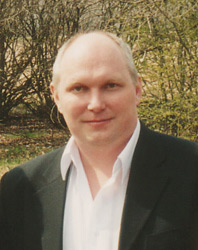a2509@yahoo.com